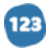# Methodology of the Naïve Bayes Algorithm.

explanatory Essay
1525 words
1525 wordsMethodology
In this chapter we are going to provide more insight into the Naïve Bayes algorithm. The aim is to show how the method works. We will also take a look at how our model will be developed, the various data sets that will be used in the process and how they were chosen. Then we are going to look at feature selection and how it will be applied.

THE NAÏVE BAYES CLASSIFIER

Bayes' rule:

P (E | H) x P (H)
P (H | E) = _________________
P (E)

The fundamental concept of Bayes' rule is that the result of a hypothesis or an event (H) can be calculated based on the presence of some observed evidences (E). From Bayes' rule, we have:
1. A prior probability of H or P(H): This is the probability of an event before observing the evidence.
2. A posterior probability of H or P(H | E): This is the probability of an event after observing the evidence.
For example to estimate the probability of a mail being classified as belonging to the Human Resources (HR) class, we usually use some evidences such as the frequency of use of words like “Employment”.

Using the equation above, let ‘HR’ be the event of a mail belonging to HR and ‘Employment’ be the evidence of the word Employment in the mail, then we have

P (Employment | HR) x P (HR)
P (HR | Employment) = _____________________
P (Employment)

P (HR | Employment) is the probability that the word Employment occurs in a mail to HR. Of course, “Employment” could occur in many other mail classes such as Joint Venture or Procurement and Contracting, but we only consider “Employment” in the context of class “HR”. This probability can be obtained from historical mail collections.
P (HR) is the prior probability of the HR class. This probability can be estimated from r...

... middle of paper ...

...st results. Because no information about the test set was used in developing the classifier, the results of this experiment should be indicative of actual performance in practice.
It is highly important to not look at the test data while developing the classifier method and to run systems on it as sparingly as possible. Ignoring or violating this rule will result in loss of validity of your results because you have implicitly tuned your system to the test data simply by running many variant systems and keeping the tweaks to the system that worked best on the test set.

Feature Selection

CONCLUSION
In this chapter we have been able to describe what the Naïve Bayes theory is and how we were able to build the classifier. In the next chapter we will take a closer look at the training set and test set. We will also carry out an evaluation of the classifier we developed.

#### In this essay, the author

• Explains how the nave bayes algorithm works, the data sets that will be used in the process, and how they were selected.
• Explains the fundamental concept of bayes' rule: the result of a hypothesis or an event can be calculated based on the presence of some observed evidences.
• Explains a prior probability of h or p(h): this is the likelihood of an event before observing the evidence.

•